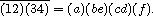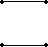Generating orbit representatives

## Generating orbit representatives

The evaluation of an orbit transversal is of limited use since these sets are usually very (for example there are approximately 109 graphs on v=11 vertices). Thus we are looking for a method which allows to examine further cases, e.g. graphs of more than ten vertices, say. In order to cover such cases with some success, we have to accept redundancy and hence we shall ran into the isomorphism disease. Invariants, isomorphism tests and all that will have to be discussed. To begin with we need to check that we can generate orbit representatives uniformly at random.

The Dixon/Wilf Algorithm If GX denotes a finite action, then we can generate orbit representatives uniformly at random in the following way:
• Choose a conjugacy class C of G with probability
p(C):=( | C | | Xg | )/( | G | | G\\X | ), where gÎC.
• Pick any gÎC and generate a fixed point of g, uniformly at random.
Then the probability that x is an element of the orbit wÎG\\X is 1/ | G\\X | , i.e. x is uniformly distributed over the orbits of G on X.

Proof: Let C1,...,Cr denote the conjugacy classes of G, with representatives giÎCi. Then, by the Cauchy-Frobenius lemma, we have

åi=1rp(Ci)=(åi | Ci | | Xgi | )/(åg | Xg | )=1,

so that p(-) defines in fact a probability distribution. If wÎG\\X, then

p(xÎw)=åip(Ci)p(xÎCiÇw)
=åip(Ci)( | XgiÇw | )/( | Xgi | ) =åi( | Ci | | Xgi | )/( | G | | G\\X | )( | XgiÇw | )/( | Xgi | )
= (1)/( | G | | G\\X | )åi | Ci | | Xgi Çw | =(1)/(åg | Xg | )åg | XgÇw | ,

where the last identity follows from exercise. Now

åg | XgÇw | =ågÎGåxÎXgÇw1= åxÎwågÎXg1=åxÎw | Gx | ,

which is equal to | Gy | | w | = | G | , for any yÎw, and we are done.

The application of this method to the generation of representatives of G-classes, H-classes, H´G-classes and H wr X G -classes on YX is easy, since we know the fixed points very well. Let us consider the G-classes, for example.

Corollary: For finite GX and Y the following procedure yields elements fÎYX that are distributed over the G-classes on YX uniformly at random:
• Choose a conjugacy class C of G with the probability
p(C):=( | C | | Y | c(bar (g')))/(åg | Y | c( bar (g))), g'ÎC.
• Pick any gÎC, evaluate its cycle decomposition and construct an fÎYX that takes values yÎY on these cycles which are distributed uniformly at random over Y.

Consider our standard example for this situation:

Example: We would like to generate graphs on 4 vertices uniformly at random. The conjugacy classes are parametrized by the proper partitions (4),(3,1),(22),(2,12) and (14) of 4. Their orders are 6,8,3,6 and 1. The corresponding numbers of cyclic factors of the permutations induced on the set [4 choose 2] of pairs of vertices are 2,2,4,4 and 6, so that the numbers of fixed points on the set 2[4 choose 2] of labeled graphs amount to 4,4,16,16 and 64. This yields for the probabilities p(C) the values
3/33,4/33,6/33,12/33,8/33.
These numbers may be multiplied by the common denominator 33 in order to get the natural numbers 3,4,6,12,8, which we accumulate obtaining
3,7,13, 25,33.
A generator that yields natural numbers between 1 and 33 uniformly at random is now used in order to choose C. As soon as it generates one of the numbers 1,2 or 3 this means that the first one of the conjugacy classes is chosen. If it generates 4,5,6 or 7, the second class is chosen, and so on. Assume that it generates 12, then the third conjugacy class is picked, an element of which is the permutation (12)(34). It induces on the set of pairs of vertices
{a={1,2},b={1,3},c={1,4},d={2,3},e={2,4},f={3,4}}
the permutationA random generator of zeros and ones is now used in order to associate values 0 or 1 with the cyclic factors of (a)(be)(cd)(f). If it generates the sequence 1,0,0,1,say, we obtain the labelled graph that has edges joining the elements of the pairs a and f, while all the other pairs of vertices remain disjoint. Its orbit is represented by the graph shown in figure.

The generated graphThis method can easily be refined in order to generate graphs with prescribed number of edges or, more generally, representatives of orbits with a given weight, uniformly at random. If GX is a finite action, w:X -> R a weight function, then G acts on the union of orbits with a prescribed weight rÎR, i.e. on the inverse image w-1[{r}] of r, and we need only to replace in the Dixon/Wilf Algorithm the corresponding factors in the numerator and in the denominator obtaining

Corollary: If we choose the conjugacy class CÍG with the probability
p(C):=( | C | | w-1[{r}]g | )/( | G | | G\\w-1[{r}] | ),
pick any element gÎC and generate a fixed point of weight r of g uniformly at random, then we obtain an element of X that is uniformly distributed over the orbits of weight r.

In the case when the acting group is a symmetric group, the choice of a conjugacy class amounts to the choice of a proper partition. We therefore should not forget that the number of proper partitions of nÎN is rapidly increasing with n. Here are a few of these numbers:

 n no. of proper partitions 10 42 20 627 40 37338 60 »10^6 100 »2·10^8

This shows that it is worthwile to try to avoid that this amount of probabilities is stored throughout the whole generating process. For practical purposes one can start the generation and evaluate probabilities only if required. This means that we need to evaluate p(Ci) only if the generated random number exceeds åj=1i-1p(Cj). The efficiency of this revised method heavily depends on the numbering of the conjugacy classes. The numbering should clearly be chosen in such a way that p(Ci)³p(Ci+1). Asymptotic considerations show (cf. Oberschelp, Dixon/Wilf) that in case of graph generation it is not bad to number the conjugacy classes of the symmetric group according to the lexicographic order of partitions.

In order to generate representatives of the orbits of G on X with prescribed type [~U] , we can do the following. As each such orbit wÎG\\X does contain elements x which have U as stabilizer, we can cut off from XU each element that has a bigger stabilizer. It clearly suffices to remove from XU the elements belonging to the XV, where U is maximal in V. The remaining subset of XU will be indicated as follows:

[^X] U:=XU\ÈU max.VXV.

The intersection of wÎX\\[~U] with [^X] U is the orbit of an xÎw, where Gx=U, under the normalizer subgroup NG(U), this is easy to check. As Gx=U we can even consider the action of the factor group NG(U)/U on this intersection wÇ[^X] U. This proves

Lemma: Each transversal of (NG(U)/U)\\[^X] U is a transversal of the orbits of type [~U] of G on X.

Moreover, if we want to apply probabilistic methods, we can use that the orbits of NG(U)/U on [^X] U are all of the same order | NG(U)/U | . Hence we obtain

Theorem:  (Laue) By picking elements from the orbits of NG(U)/U on [^X] U uniformly at random, we obtain elements that are uniformly distributed over the orbits of type [~U] of G on X.

For example, if U:={1}, we have that [^X] U=[^X] 1 is equal to X minus the union of the fixed point sets XV, taken over all the minimal subgroups V£G. If in particular G:=Cn, then the minimal subgroups V are just the cyclic subgroups Cp£Cn, where p is prime. the same holds for G:=Sn , but in this case there may be, of course, several such Cp. As NG(1)=G, we can, according to Laue's theorem, easily generate representatives of the orbits of maximal length | bar (G) | , uniformly at random. In order to display a concrete example, we recall the enumeration of primitive polynomials of degree n over GF(q) (recall example). They correspond to the orbits of maximal length n of the Galois group Cn of GF(q)n over GF(q). The above reasoning shows that an orbit of G=NG(1) on [^X] 1 is also a transversal of these orbits of maximal length n of G on X. But [^X] 1 is equal to GF(q)n minus the set of fixed points GF(q)Cp, where p runs through the prime divisors of n. These subsets which have to be subtracted, can easily be spotted. For example, if n:=6,q:=2, C6:=á(1...6)ñ, we obtain

GF(2)6C2={000000,100100,010010,
001001,110110,101101, 011011,111111},
GF(2)6C3={000000,101010,010101,111111}.

Subtracting the union of these sets from GF(2)6, we get [^X] 1, consisting of the remaining 26-10 binary sequences over GF(2). A transversal of the orbits of C6 on [^X] 1 is, for example. the set

100000,110000,101000,10010,111000,110100,110010,111100,111110.

Assuming a normal basis given, we can translate these sequences into the 9 corresponding polynomials that are listed in many books.

Exercises
E: Prove that, for conjugate g,g'ÎG, a finite action GX and an orbit wÎG\\X, we have
| XgÇw | = | Xg'Çw | .

 harald.fripertinger "at" uni-graz.at http://www-ang.kfunigraz.ac.at/~fripert/ UNI-Graz Institut für Mathematik UNI-Bayreuth Lehrstuhl II für Mathematik
last changed: January 19, 2005Generating orbit representatives